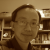3n + 1 conjectureFarewell 2021 with 3n+1

The Youtube algorithm one day pushed a video "The Simplest Math Problem No One Can Solve - Collatz Conjecture" to me. After viewing the video, I thought that my VRMath2 could easily visualise the 3n+1 graph with its LOGO programing. I am happy that my VRMath2 application is still working after almost three years in low maintenance mode. With some simple coding. I have written a LOGO program that can take a number (natural number) and the turtle will draw its 3n+1 graph. The 3n+1 problem is simple. It starts with a natural number; if the number is odd, multiply it by 3 then add one; if the number is even, the number is divided by 2. These rules apply until the resulting number is one. It is interesting that it seems any given natural number will end with 1 after applying the 3n+1 rules, but how?